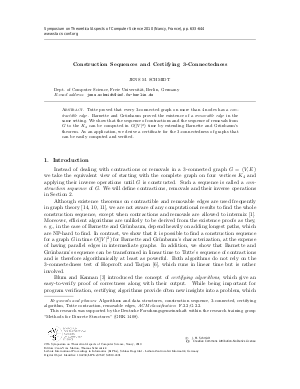Document# Construction Sequences and Certifying 3-Connectedness

### Author Jens M. Schmidt## File

LIPIcs.STACS.2010.2491.pdf
• Filesize: 314 kB
• 12 pages

## Cite As

Jens M. Schmidt. Construction Sequences and Certifying 3-Connectedness. In 27th International Symposium on Theoretical Aspects of Computer Science. Leibniz International Proceedings in Informatics (LIPIcs), Volume 5, pp. 633-644, Schloss Dagstuhl - Leibniz-Zentrum für Informatik (2010)
https://doi.org/10.4230/LIPIcs.STACS.2010.2491

## Abstract

Given two $3$-connected graphs $G$ and $H$, a \emph{construction sequence} constructs $G$ from $H$ (e.\,g. from the $K_4$) with three basic operations, called the \emph{Barnette-Gr\"unbaum operations}. These operations are known to be able to construct all $3$-connected graphs. We extend this result by identifying every intermediate graph in the construction sequence with a subdivision in $G$ and showing under some minor assumptions that there is still a construction sequence to $G$ when we start from an \emph{arbitrary prescribed} $H$-subdivision. This leads to the first algorithm that computes a construction sequence in time $O(|V(G)|^2)$. As an application, we develop a certificate for the $3$-connectedness of graphs that can be easily computed and verified. Based on this, a certifying test on $3$-connectedness is designed.%Finding certifying algorithms is a major goal for problems where the efficient solutions known are complicated. Tutte proved that every $3$-connected graph on more than $4$ nodes has a \emph{contractible edge}. Barnette and Gr\"unbaum proved the existence of a \emph{removable edge} in the same setting. We show that the sequence of contractions and the sequence of removals from $G$ to the $K_4$ can be computed in $O(|V|^2)$ time by extending Barnette and Gr\"unbaum's theorem. As an application, we derive a certificate for the $3$-connectedness of graphs that can be easily computed and verified.
##### Keywords
• Construction sequence
• 3-connected graph
• nested subdivisions
• inductive characterization
• 3-connectedness
• certifying algorithm

## Metrics

• Access Statistics
• Total Accesses (updated on a weekly basis)
0